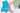We all know that in layman’s terms the rate of inflation measures the general level of price increases over a given period of time. But the above quoted figure is more precisely known as the Consumer Price Index (CPI).

Unfortunately I have not been afforded the luxury of word-count to explain the long list of different inflation measures, of which I can think of at least nine. What I will try to do is briefly explain the main differences between the most quoted measures; the Retail Price Index (RPI) and the previously mentioned CPI.

The basic methodological approach for both the CPI and the RPI is the same, in that they both track roughly 180,000 individual prices for over 650 goods and services.

One of the differences though is in the constituents where the RPI includes housing costs, such as mortgage interest payments and council tax. Aside from 2008 and the obvious drop in mortgage rates, why do we see RPI constantly print above CPI then?

This is the result of the other, more important difference, which is in the calculation where the RPI measures the arithmetic mean (add up the total prices and divide by the number of goods). Whilst the CPI uses the geometric mean (multiply prices of individual goods and take the nth root), which mathematically will produce an average less than or equal to the arithmetic mean. This calculation differential is also known as the formula effect and accounts for around a 0.9% difference between the RPI and the CPI.

So if your children start asking that, given the recent drop in sterling, their pocket money becomes inflation-linked, remember to include in the small print that you intend on using a geometric mean of prices.